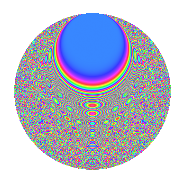# Properties

 Label 8039.2.aLevel 8039 Weight 2 Character orbit a Rep. character $$\chi_{8039}(1,\cdot)$$ Character field $$\Q$$ Dimension 670 Newforms 2 Sturm bound 1340 Trace bound 1

# Related objects

## Defining parameters

 Level: $$N$$ = $$8039$$ Weight: $$k$$ = $$2$$ Character orbit: $$[\chi]$$ = 8039.a (trivial) Character field: $$\Q$$ Newforms: $$2$$ Sturm bound: $$1340$$ Trace bound: $$1$$

## Dimensions

The following table gives the dimensions of various subspaces of $$M_{2}(\Gamma_0(8039))$$.

Total New Old
Modular forms 671 671 0
Cusp forms 670 670 0
Eisenstein series 1 1 0

The following table gives the dimensions of the cuspidal new subspaces with specified eigenvalues for the Atkin-Lehner operators.

$$8039$$Dim.
$$+$$$$279$$
$$-$$$$391$$

## Trace form

 $$670q$$ $$\mathstrut +\mathstrut q^{2}$$ $$\mathstrut +\mathstrut 673q^{4}$$ $$\mathstrut +\mathstrut 2q^{5}$$ $$\mathstrut +\mathstrut 6q^{7}$$ $$\mathstrut -\mathstrut 3q^{8}$$ $$\mathstrut +\mathstrut 676q^{9}$$ $$\mathstrut +\mathstrut O(q^{10})$$ $$670q$$ $$\mathstrut +\mathstrut q^{2}$$ $$\mathstrut +\mathstrut 673q^{4}$$ $$\mathstrut +\mathstrut 2q^{5}$$ $$\mathstrut +\mathstrut 6q^{7}$$ $$\mathstrut -\mathstrut 3q^{8}$$ $$\mathstrut +\mathstrut 676q^{9}$$ $$\mathstrut -\mathstrut 2q^{10}$$ $$\mathstrut +\mathstrut 4q^{11}$$ $$\mathstrut -\mathstrut 16q^{12}$$ $$\mathstrut +\mathstrut 8q^{13}$$ $$\mathstrut -\mathstrut 10q^{14}$$ $$\mathstrut +\mathstrut 675q^{16}$$ $$\mathstrut +\mathstrut 4q^{17}$$ $$\mathstrut -\mathstrut 3q^{18}$$ $$\mathstrut +\mathstrut 18q^{19}$$ $$\mathstrut -\mathstrut 8q^{20}$$ $$\mathstrut +\mathstrut 18q^{21}$$ $$\mathstrut +\mathstrut 16q^{22}$$ $$\mathstrut +\mathstrut 4q^{23}$$ $$\mathstrut +\mathstrut 4q^{24}$$ $$\mathstrut +\mathstrut 684q^{25}$$ $$\mathstrut +\mathstrut 12q^{26}$$ $$\mathstrut -\mathstrut 18q^{27}$$ $$\mathstrut +\mathstrut 36q^{28}$$ $$\mathstrut +\mathstrut 10q^{29}$$ $$\mathstrut +\mathstrut 16q^{30}$$ $$\mathstrut +\mathstrut 4q^{31}$$ $$\mathstrut +\mathstrut 5q^{32}$$ $$\mathstrut +\mathstrut 14q^{33}$$ $$\mathstrut +\mathstrut 22q^{34}$$ $$\mathstrut -\mathstrut 18q^{35}$$ $$\mathstrut +\mathstrut 721q^{36}$$ $$\mathstrut +\mathstrut 12q^{37}$$ $$\mathstrut +\mathstrut 2q^{38}$$ $$\mathstrut +\mathstrut 4q^{39}$$ $$\mathstrut +\mathstrut 16q^{40}$$ $$\mathstrut +\mathstrut 12q^{41}$$ $$\mathstrut +\mathstrut 26q^{42}$$ $$\mathstrut +\mathstrut 14q^{43}$$ $$\mathstrut +\mathstrut 20q^{44}$$ $$\mathstrut -\mathstrut 8q^{45}$$ $$\mathstrut +\mathstrut 62q^{46}$$ $$\mathstrut -\mathstrut 20q^{47}$$ $$\mathstrut -\mathstrut 22q^{48}$$ $$\mathstrut +\mathstrut 690q^{49}$$ $$\mathstrut -\mathstrut 11q^{50}$$ $$\mathstrut +\mathstrut 12q^{51}$$ $$\mathstrut +\mathstrut 16q^{52}$$ $$\mathstrut -\mathstrut 6q^{53}$$ $$\mathstrut +\mathstrut 14q^{54}$$ $$\mathstrut +\mathstrut 2q^{55}$$ $$\mathstrut -\mathstrut 22q^{56}$$ $$\mathstrut +\mathstrut 44q^{57}$$ $$\mathstrut +\mathstrut 16q^{58}$$ $$\mathstrut -\mathstrut 18q^{59}$$ $$\mathstrut -\mathstrut 18q^{60}$$ $$\mathstrut +\mathstrut 32q^{61}$$ $$\mathstrut +\mathstrut 12q^{62}$$ $$\mathstrut -\mathstrut 2q^{63}$$ $$\mathstrut +\mathstrut 683q^{64}$$ $$\mathstrut +\mathstrut 30q^{65}$$ $$\mathstrut +\mathstrut 2q^{66}$$ $$\mathstrut +\mathstrut 22q^{67}$$ $$\mathstrut -\mathstrut 42q^{68}$$ $$\mathstrut -\mathstrut 14q^{69}$$ $$\mathstrut -\mathstrut 20q^{70}$$ $$\mathstrut -\mathstrut 12q^{71}$$ $$\mathstrut -\mathstrut 15q^{72}$$ $$\mathstrut +\mathstrut 42q^{73}$$ $$\mathstrut -\mathstrut 38q^{74}$$ $$\mathstrut -\mathstrut 34q^{75}$$ $$\mathstrut +\mathstrut 60q^{76}$$ $$\mathstrut +\mathstrut 30q^{77}$$ $$\mathstrut -\mathstrut 38q^{78}$$ $$\mathstrut +\mathstrut 42q^{79}$$ $$\mathstrut -\mathstrut 26q^{80}$$ $$\mathstrut +\mathstrut 718q^{81}$$ $$\mathstrut -\mathstrut 32q^{82}$$ $$\mathstrut +\mathstrut 20q^{83}$$ $$\mathstrut +\mathstrut 88q^{84}$$ $$\mathstrut +\mathstrut 70q^{85}$$ $$\mathstrut +\mathstrut 18q^{86}$$ $$\mathstrut +\mathstrut 14q^{87}$$ $$\mathstrut +\mathstrut 8q^{88}$$ $$\mathstrut +\mathstrut 22q^{89}$$ $$\mathstrut -\mathstrut 46q^{90}$$ $$\mathstrut +\mathstrut 26q^{91}$$ $$\mathstrut -\mathstrut 36q^{92}$$ $$\mathstrut +\mathstrut 16q^{93}$$ $$\mathstrut -\mathstrut 4q^{94}$$ $$\mathstrut -\mathstrut 26q^{95}$$ $$\mathstrut +\mathstrut 14q^{96}$$ $$\mathstrut +\mathstrut 42q^{97}$$ $$\mathstrut +\mathstrut 13q^{98}$$ $$\mathstrut +\mathstrut 92q^{99}$$ $$\mathstrut +\mathstrut O(q^{100})$$

## Decomposition of $$S_{2}^{\mathrm{new}}(\Gamma_0(8039))$$ into irreducible Hecke orbits

Label Dim. $$A$$ Field CM Traces A-L signs $q$-expansion
$$a_2$$ $$a_3$$ $$a_5$$ $$a_7$$ 8039
8039.2.a.a $$279$$ $$64.192$$ None $$-13$$ $$-12$$ $$-20$$ $$-57$$ $$+$$
8039.2.a.b $$391$$ $$64.192$$ None $$14$$ $$12$$ $$22$$ $$63$$ $$-$$